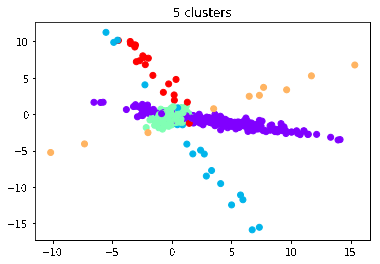### Model Preliminaries

1. Dirichlet Process(DP) is a stochastic process used in Bayesian nonparametrics, particularly in Dirichlet Process mixture models(DPMM) (aka infinite mixture models).
2. The DP is a distribution over distributions, i.e, each draw, $G$, from the DP is a distribution.

We first generate data from the Dirichlet Process: \

• Generate a sample distribution $G \sim DP(\alpha, G_0)$, where $\alpha$ is the concentration measure and $G_0$ is the “base measure”.

• A sample from the Dirichlet Process is a “distribution over cluster distributions” given by

\begin{equation} G=\sum_{k=1}^{\infty}\rho_k \delta_{\mu_k} \end{equation}

• The probabilities or proportions for each cluster $k$ is given by $\rho_k \sim GEM(\alpha)$ (stick-breaking), and the cluster parameters $\mu_k \sim G_0$. Our data are generated by: $x_i \sim \mathcal{N}(\mu_k, \sigma^2)$

• Notice that there is an $\infty$ in the above equation. We dont actually make the computer generate an infinite number of clusters. Instead the key idea is to generate new clusters with some probability each time we sample a new datapoint, so theoretically, there is no bound on the number of clusters we could have if $n\rightarrow \infty$. Clearly, we must be doing something with the probabilities such that they don’t explode and still sum to 1. This process is known as “stick-breaking”, where the “stick” represents $\sum_{k=1}^{\infty}\rho_k = 1$.

• For $k=1, \rho_1 = \beta (1, \alpha)$
• For $k=2, \rho_2 = \beta (1, \alpha) * (1-\rho_1)$
• For $k=3, \rho_3 = \beta (1, \alpha) * (1-\rho_1-\rho_2)$
• Later $\rho_k$ gets a shorter and shorter end of the stick. By always taking a portion of what’s remaining, this ensures that the sum of $p_k$’s is still 1

• $\alpha$ and $G_0$ are hyperparameters. The base distribution, $G_0$, is basically the “mean” of the DP. For any measurable set $A \subset \Theta$, $E[G(A)] = G_0(A)$. $\alpha$ can be thought of as an inverse variance, the larger the $\alpha$, the smaller the variance, i.e, a draw from the DP will be more concentrated around the base distribution.

The generation of $\mu_k$ comes from our base measure, which is a completely separate process to the stick-breaking described above. Since we are modeling a multivariate Gaussian (2-dimensions) mixture model, we choose a conjugate prior for $G_0$ for analytical convenience, i.e. a Normal distribution parameterised by a mean and covariance matrix. We will use an inverse wishart distribution, parameterised with degrees of freedom, $\nu$, equal to the number of dimensions, and let $\Psi$ be an identity matrix to generate the $\Sigma_k$ generating $\mu_k$.

\begin{align} \Sigma_k &\sim \mathcal{W}^{-1}(\nu, \Psi) \\ \mu_k &\sim \mathcal{N}(\mu_0, \Sigma_k) \end{align}

We can now generate our data from the cluster assignments and our stored cluster parameters.

Each time we sample a new $G$, we get a different distribution and therefore a different number of clusters: